# Иностранная литература и современность: General Statistics: Ch 2 Test Flashcards

Set Details Share
created 9 years ago by GreenHero64
28,209 views
This course is designed to acquaint the student with the principles of descriptive and inferential statistics. Topics will include: types of data, frequency distributions and histograms, measures of central tendency, measures of variation, probability, probability distributions including binomial, normal probability and student's t distributions, standard scores, confidence intervals, hypothesis testing, correlation, and linear regression analysis. This course is open to any student interested in general statistics and it will include applications pertaining to students majoring in athletic training, pre-nursing and business.
updated 9 years ago by GreenHero64
College: First year, College: Second year, College: Third year, College: Fourth year
Subjects:
statistics, mathematics, probability & statistics
Page to share:
Embed this setcancel
COPY
code changes based on your size selection
Size:
X

1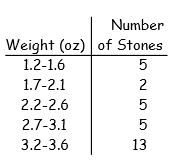Construct the cumulative frequency distribution that corresponds to the given frequency distribution.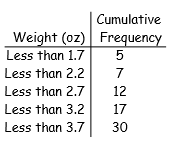5 (+5)

7 (5 + 2)

12 (5 + 2 + 5)

17 (5 + 2 + 5+ 5)

30 (5 + 2 + 5 + 5 + 13)

2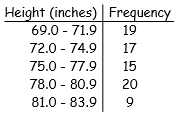Construct the cumulative frequency distribution that corresponds to the given frequency distribution.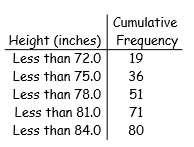19 (+19)

36 (19 + 17)

51 (19 + 17 + 15)

71 (19 + 17 + 15 + 20)

80 (19 + 17 + 15 + 20 + 9)

3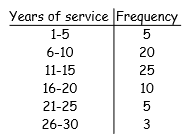Provide an appropriate response.

The frequency distribution below summarizes employee years of service for Alpha Corporation.

Find the class boundaries for class 26-30.

25.5, 30.5

4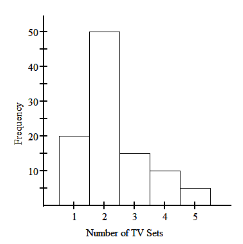Provide an appropriate response.

The histogram below represents the number of television sets per household for a sample of U.S.households.

How many households are included in the histogram?

100

sum total of frequencies

= (20 + 50 + 15 + 10 + 5)

5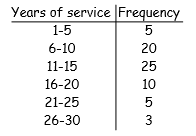Provide an appropriate response.

The frequency distribution below summarizes employee years of service for Alpha Corporation.

Find the class midpoint for class 1-5.

3.0

midpoint = (1 + 5) ÷ 2 = 3.0

6

Provide an appropriate response.

Sturges' guideline suggests that when constructing a frequency distribution, the ideal number of classes can be approximated by 1 + (log n)/(log 2), where n is the number of data values.

Use this guideline to find the ideal number of classes when the number of data values is 146.

8

1 + ( log(146) ) ÷ ( log(2) )

= 8.2

7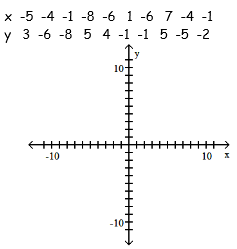Use the given paired data to construct a scatterplot.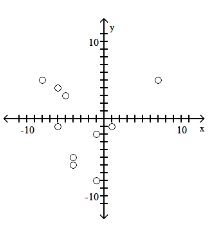8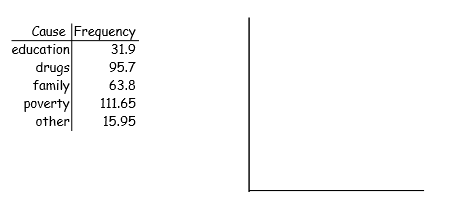Solve the problem.

At the National Criminologists Association's annual convention, participants filled out a questionnaire asking what they thought was the most important cause for criminal behavior. The tally was as follows.

Construct a Pareto chart to display these findings.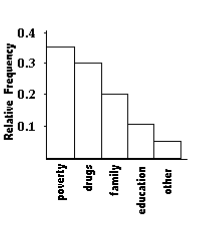9

Construct the dotplot for the given data.

A store manager counts the number of customers who make a purchase in his store each day. The data are as follows.

15 16 13 19 12 15 15 16 13 12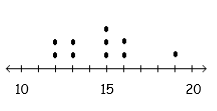10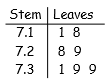Find the original data from the stemplot.

7.11, 7.18, 7.28, 7.29, 7.31, 7.39, 7.39